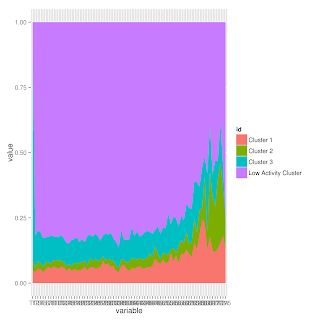# Roles Visualization

less than 1 minute read

Published:

So, I thanks to some help from the very kind BrodieG on Stack Overflow, I was finally able to get some visualizations of the way that roles change over time. I am using the following code (as you can see, I tried to learn how to do melting and reshaping, then kind of gave up that hope - maybe another time).

`library(reshape2)library(ggplot2)#clusters.mlt <- melt(clusters, id.vars="id")#clusters.agg <- aggregate(. ~ id + variable, clusters.mlt, sum)# The minimum number of times a user has to be in a given group in order to# be shown in the graph for that groupminMonths = 2makeGraph <- function(clusters){        clus1 <- apply(clusters, 2, function(x) {sum(x=='1', na.rm=TRUE)})        clus2 <- apply(clusters, 2, function(x) {sum(x=='2', na.rm=TRUE)})        clus3 <- apply(clusters, 2, function(x) {sum(x=='3', na.rm=TRUE)})        clus0 <- apply(clusters, 2, function(x) {sum(x=='0', na.rm=TRUE)})        clusters2 <- data.frame(clus0, clus1, clus2, clus3)        c2 <- t(clusters2)        c3 <- as.data.frame(c2)        c3\$id = c('Low Activity Cluster', 'Cluster 1', 'Cluster 2', 'Cluster 3')        c3 <- c3[order(c3\$'id'),]        return(ggplot(melt(c3, id.vars="id")) +          geom_area(aes(x=variable, y=value, fill=id, group=id), position="fill"))}#print(ggplot(clusters.mlt) + # stat_summary(aes(x=variable, y=value, fill=id, group=id), fun.y=sum, position="fill",        geom="area"))# Stats for just those who were in each groupclusterDF <- as.data.frame(read.csv('clustersByID.csv'))ggsave(file="../Results/allUsers.png", plot=makeGraph(clusterDF))cl1 <- clusterDF[apply(clusterDF, 1, function(x) {sum(x[2:76] == "1", na.rm=TRUE) >=            minMonths}),]ggsave("../Results/Role1_2+.png", makeGraph(cl1))`
And this is what the code produces (some new colors would probably be a good thing to work on next!)There are some interesting things going on here, but no clear movement into the central-type role (Role 1).